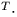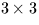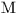Next: SLA_DJCAL - MJD to Gregorian for Output
Up: SUBPROGRAM SPECIFICATIONS
Previous: SLA_DH2E - Az,El to

## SLA_DIMXV - Apply 3D Reverse Rotation

ACTION:
Multiply a 3-vector by the inverse of a rotation matrix (double precision).

CALL:
CALL sla_DIMXV (DM, VA, VB)

GIVEN:

 D(3,3) DM rotation matrix VA D(3) vector to be rotated

RETURNED:

 D(3) VB result vector

NOTES:
1.
This routine performs the operation:
b = Ma
where a and b are the 3-vectors VA and VB respectively, and M is thematrix DM.
2.
The main function of this routine is apply an inverse rotation; under these circumstances,is orthogonal, with its inverse the same as its transpose.
3.
To comply with the ANSI Fortran 77 standard, VA and VB must not be the same array. The routine is, in fact, coded so as to work properly on the VAX and many other systems even if this rule is violated, something that is not, however, recommended.Next: SLA_DJCAL - MJD to Gregorian for Output
Up: SUBPROGRAM SPECIFICATIONS
Previous: SLA_DH2E - Az,El to

SLALIB --- Positional Astronomy Library